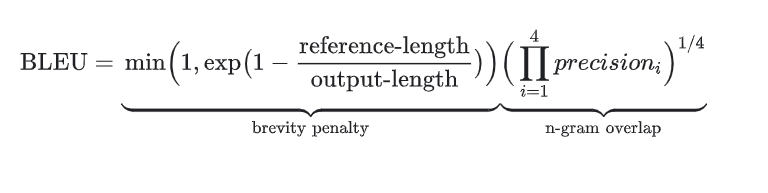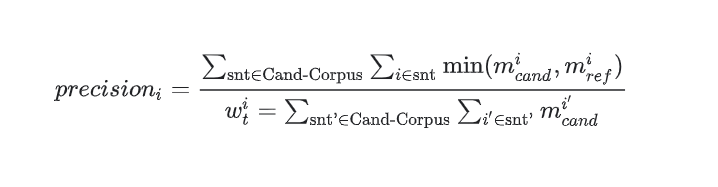×

Search anything:

# Understanding Bleu Score

#### Machine Learning (ML) Natural Language Processing (NLP)Get this book -> Problems on Array: For Interviews and Competitive Programming

BLEU (Bilingual Evaluation Understudy) score was proposed in 2002 and has quickly become the standard score for evaluating machine translation output. It measures the similarity between a machine's translated text and a set of good quality human reference translations.

I. Why is BLEU score important?
II. How is BLEU score calculated?
III. Mathematical Implementation
IV. Code Implementation
V. Advantages and Limitations of BLEU score
VI. Similar evaluation metrics
VII. Applications of BLEU score

## Why is BLEU score important?

Machine translation is the task of automatically translating text from one language to another using computer software. A key challenge in machine translation is evaluating the quality of the translated output and BLEU score is one of the most popular and effective automatic evaluation metrics.

BLEU score is calculated for individual translated segments, such as sentences in a paragraph of translated text. It counts the number of overlapping words between the generated translation and the reference translations. Larger overlap indicates better translation quality.

## How is BLEU Score calculated?

The BLEU metric ranges from 0 to 1.

• 0 indicates completely dissimilar translations while 1 indicates identical translations.

The key steps to calculate BLEU are:

1. Count the number of matching n-grams between the candidate and reference translations, here N-grams depict sequences of n consecutive words in a given sentence.
2. Calculate a precision score for each n-gram length (e.g. unigrams, bigrams, trigrams, etc).
3. Use those precision scores to calculate a brevity penalty that penalizes short translations.
4. Compute the geometric mean of the modified n-gram precisions and brevity penalty to get the final BLEU score.
5. Higher order n-grams (e.g. trigrams, 4-grams) account for fluency while lower order n-grams (e.g. unigrams, bigrams) account for adequacy of translation. The brevity penalty prevents inflated BLEU scores for short translations.

## Mathematical Implementation

The mathematical formula to calculate BLEU score is given as:The formula contains two parts as depicted above:

1. Brevity Penalty applies a penalty to the generated translations that are too short compared to the reference translations. This compensates for BLEU score not having a recall term which identifies the number of generated translations that are true to the reference translations.
2. N-Gram overlap counts the number of word sequences in generated text that correctly match the word sequences in the reference text. This is basically a precision metric for BLEU score.

In BLEU Score, the precision is given by:So, how do we use these formulas to calculate the BLEU score?

• Consider two sentences, a reference sentence, or the original sentence, and a machine-generated candidate sentence.
``````Reference (r) = "the cat is on the mat"
Candidate (c) = "the the the cat mat"
``````
• The first step is to calculate the n-gram precision, for this example, we will stick to unigram precision, which determines how accurately each word has been generated.
``````Unigrams in reference sentence = 6 (“the”, “cat”, “is”, “on”, “the”, “mat”)
Unigrams in machine-generated sentence = 5 (“the”, “cat”, “cat”, “cat”, “mat”)
Matching unigrams = 3 (“the”, “cat”, “mat”)
Unigram precision = 3/5 = 0.6
``````
• Now, we calculate the brevity penalty:
``````Length of reference sentence (r) = 6
Length of machine-generated sentence (c) = 5

If c <= r : BP = 1
If c > r : BP = exp(1-r/c)

Since the length of the candidate sentence is less than that of the reference,
BP = 1
``````
• Finally, the BLEU score can be calculated as:
``````BLEU score = BP * uni-gram precision = 1 * 0.6

Therefore,
BLEU score = 0.6
``````

## Code Implementation

BLEU Score can be calculated for two sentences using the following example:

• Note that the BLEU score is case-sensitive.
``````import nltk
from nltk.translate.bleu_score import sentence_bleu

# Original sentence
reference = "the cat is on the mat".split()

# Machine generated/translated sentence
candidate = "the the the cat mat".split()

score = sentence_bleu(reference, candidate)
print(score)
``````
• It is important to note that for longer sentences, more n-grams, i.e. sequences of two or more words, are compared for better precision, an example of the application of BLEU score on longer sentences is given here: BLEU Score documentation

1. Simple and fast to calculate.
2. Programming language independent.
3. High correlation with human judgment.
4. Standardized metric for comparison.

## Limitations of BLEU score

1. Only measures precision, not recall.
2. Focuses on lexical similarity, not semantic similarity.
3. Requires high quality human reference translations.
4. Not a good indicator of true quality for translations with low score.

## Comparison with similar metrics

Apart from BLEU score, there exist ROUGE (Recall Oriented Understudy for Gisting Evaluation) score and SacreBLEU score. They all serve the purpose of evaluating machine-generated and translated text but have some key differences.

### ROUGE Score

Unlike BLEU score, ROUGE score is recall oriented, making it useful for tasks where recall is more important, such as text summarization. But it has limited scope as ROUGE also doesn’t consider semantic meaning similar to BLEU.

### SacreBLEU

Compared to BLEU, SacreBLEU provides a standard way to compute BLEU scores, which helps in comparing results across different studies, making it easy to implement in Python to use in modern machine translation systems. But, as an extension of BLEU score, SacreBLEU inherits all the limitations of the BLEU score.

## Applications of BLEU score

Despite some limitations, BLEU remains the most popular metric to evaluate and benchmark machine translation output automatically. Some applications of BLEU score are:

1. Comparing translation quality of different language models.
2. Tuning hyperparameters of language models.
3. Tracking training progress of language models.
4. Evaluating human translations.

With this OpenGenus.org article, you must have a strong idea of BLEU (Bilingual Evaluation Understudy) score.

Understanding Bleu Score